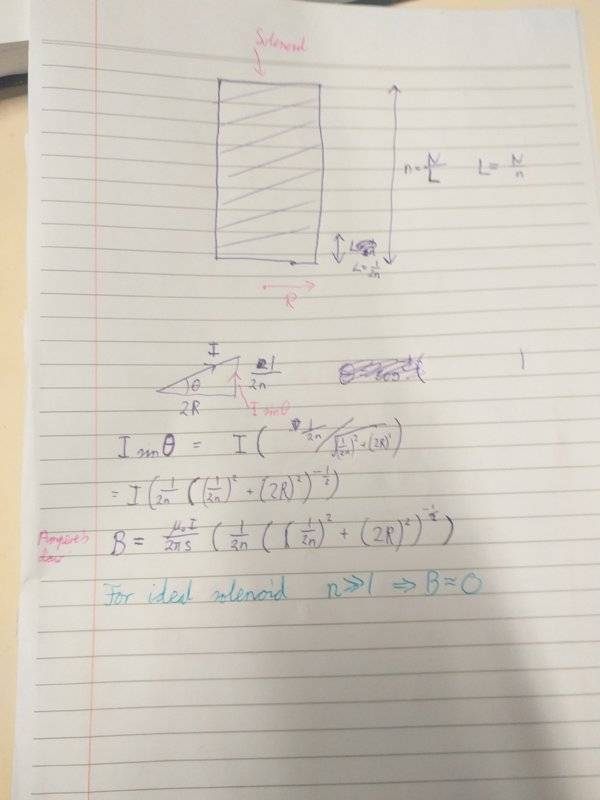# Magnetic field outside a solenoid

In his book on electrodynamics, Griffith talks about the magnetic field outside a solenoid. Firstly instead of dealing with a typical solenoid with closely wound loops, he instead works with a cylinder with a surface current that has no z-component. To get the angular component of the B-field outside the solenoid he takes an Amperian loop that is concentric with the solenoid. Then he says that no current flows through the loop and therefore due to symmetry the angular component is 0. I would like to know why he chose to work with a cylinder instead of a typical solenoid. I attached screenshots from the book below.

#### Attachments

•Delta2

Homework Helper
Gold Member
2020 Award
The magnetic surface currents from a cylinder of uniform magnetization have the same geometry as the currents of a solenoid. In practice, any solenoid will also have a current ## I ## going in the ## z ## direction along its axis, but this is usually ignored in any textbook treatment of the magnetic field of a solenoid. You are correct=this will generate a ## \phi ## component of the magnetic field, but it is normally ignored.## \\ ## (Edit: There will, in general, also be a magnetic field generated by the current in the wires leading to the solenoid, but these are also not included in the computation, since they will be depend on the particular geometry). ## \\ ## For Griffith's choice of a magnetized cylinder, perhaps he wanted to introduce the reader to the concept of a permanent magnet that has a cylindrical shape. It can be modeled as having uniform magnetization. There is also a "pole" model for computing the magnetic field ## B ## from the magnetized cylinder, which gets identical results as the magnetic surface current computation. See https://www.physicsforums.com/threads/a-magnetostatics-problem-of-interest-2.971045/

Last edited:
•Delta2
jtbell
Mentor
For Griffith's choice of a magnetized cylinder, perhaps he wanted to introduce the reader to the concept of a permanent magnet that has a cylindrical shape.
The example shown by the OP is from Griffiths (3rd ed.) Chapter 5, which doesn't discuss magnetization at all. He discusses bound currents and magnetization in Chapter 6, where he leaves the uniformly magnetized (solid) cylinder as an exercise (Problem 6.7). Therefore introducing magnetization here is "jumping the gun", so to speak.
instead of dealing with a typical solenoid with closely wound loops, he instead works with a cylinder with a surface current that has no z-component. [...] I would like to know why he chose to work with a cylinder instead of a typical solenoid.
In introductory textbooks, one normally uses simplified idealized situations to introduce applications of basic laws such as Ampère's Law.

The ##z##-component of the current does produce a ##\phi##-component of the magnetic field. It should be an easy exercise to calculate the magnetic field produced by a very long ("infinite") cylindrical shell of current in the ##z##-direction. Then combine it with the field produced by a pure "##\phi##-current", in some proportion that depends on the angle that the solenoid wires make with the ##\phi##-direction, which in turn depends on the radius of the solenoid and the number of turns of wire per unit length along the solenoid.

I'm a bit surprised that Griffiths apparently doesn't discuss this later, or give this as an exercise.

Last edited:
•Homework Helper
Gold Member
2020 Award
The example shown by the OP is from Griffiths (3rd ed.) Chapter 5, which doesn't discuss magnetization at all. He discusses bound currents and magnetization in Chapter 6, where he leaves the uniformly magnetized (solid) cylinder as an exercise (Problem 6.7). Therefore introducing magnetization here is "jumping the gun", so to speak.
@jtbell I don't have a copy of that textbook, so I may have made a premature conclusion, but thank you for the input. ## \\ ## For your second paragraph, surface current per unit length ##K=nI ##, where ## n ## is the number of turns per unit length, gives the current ## I =\frac{K}{n}## that would flow in the ## z ## direction.

The ##z##-component of the current does produce a ##\phi##-component of the magnetic field. It should be an easy exercise to calculate the magnetic field produced by a very long ("infinite") cylindrical shell of current in the ##z##-direction. Then combine it with the field produced by a pure "##\phi##-current", in some proportion that depends on the angle that the solenoid wires make with the ##\phi##-direction, which in turn depends on the radius of the solenoid and the number of turns of wire per unit length along the solenoid.

I attempted it but I wanna ask whether I'm going about it the right way:Also is the enclosed current angle always dependent? On one hand we have that:

##\oint####B.dl=####\mu_o####\int####J.da##

Which reduces to:

##\oint####B.dl=####\mu_o####I##

The first equation is a dot product between J and the area so there is a dependence on the angle. The second formula has is in terms of the current going through the surface, which as far as I know shouldn't depend on the angle. Is there anything wrong with my reasoning here?